Next: The Pairing Mechanism Up: Magnetic Properties of Conventional Superconductors Previous: BCS Theory for Conventional Superconductors

## Type-II Superconductors and the Vortex State

As presented in their original form, the above results pertain almost exclusively to type-I superconductors. The main topic of interest here however, is the magnetic properties of type-II superconductors, whose magnetization curve is depicted in Fig. 2.4. Below Tc, in the presence of an externally applied magnetic field H, three distinct phases are recognizable, dependent on the strength of the applied field. Below a lower critical field Hc1 (T)the superconductor is in the Meissner state with full expulsion of magnetic flux from its interior. For an applied field above an upper critical field Hc2 (T), magnetic flux fully penetrates the type-II material and returns it to its normal state. Within Ginzburg-Landau theory, the lower and upper critical fields may be written as: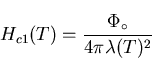(20)

and,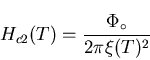(21)

respectively.

If the applied field lies between Hc1 (T)and Hc2 (T), there is a partial penetration of flux into the sample leading to regions in the interior which are superconducting and others which are in the normal state; this is often referred to as the mixed state. Ginzburg and Landau defined type-II superconductors as those with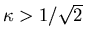. The high-temperature superconductors are extreme type-II, with large GL parameters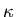(i.e.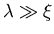), large upper critical fields Hc2 (T), and small lower critcal fields Hc1 (T) [32,33,34].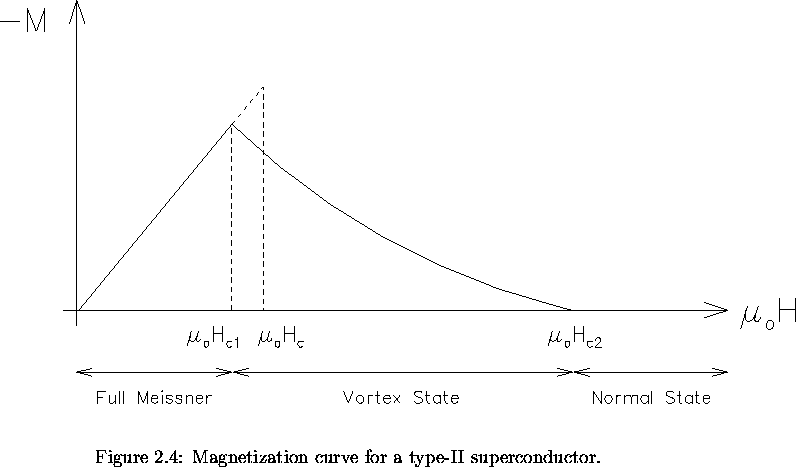In 1957, the Russian physicist Alexei A. Abrikosov predicted the existence of type-II superconductors by considering the solution of the GL equations for. In particular, he considered the case where the externally applied magnetic field H is only slightly below Hc2 (T); for in this region one obtains approximate solutions resembling those of the linearized GL equations . The solutions revealed the presence of a periodic microscopic magnetic field distribution, transverse to the applied field. More precisely his efforts predicted a periodic square array of thin filaments of magnetic flux in the mixed state. Consequently, literature sometimes refers to the mixed state as the Abrikosov regime or for reasons to soon be made apparent, the vortex state.

In the core region of a filament, the magnetic field is high and the material does not superconduct here; that is these regions are in the normal state. The magnetic field is screened from the rest of the sample by supercurrents which circulate around each filament. It is common to refer to the filaments as vortex lines and the array of filaments as a vortex lattice. At the vortex center where the magnitude of the local field is largest, the density of superconducting electrons ns and hence the order parameter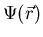is zero. As one moves radially out from the center of a vortex core, nsincreases and the supercurrents screen a greater amount of flux. At a radius on the order of the coherence length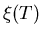, ns approaches the value in the bulk of the sample. It follows that the density of the supercurrents is greatest near the edge of the vortex core where the screening of the local magnetic field is at a maximum. Outside of the vortex core, magnetic field leaks into the superconducting regions of the sample ( see Fig. 2.5), in much the same way magnetic field penetrates the surface of the semi-infinite superconductor in Figure 1.2. The superconducting order parameter is, for all intents and purposes, constant beyond regions of the orderaround the vortex cores . It may be noted here that Abrikosov's prediction of the vortex state was remarkable in that it precluded any concrete experimenital proof of its existence. He derived the following relations between Hc1, Hc2, and the thermodynamic critical field Hc: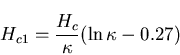(22)

and,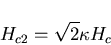(23)

At a field Hc2 the vortex cores begin to overlap and there is no longer any solution of the Ginzburg-Landau equation (i.e. the material returns to its normal state) . Hc1 marks the magnetic field at which it first becomes energetically favourable to have a flux line penetrate the superonductor.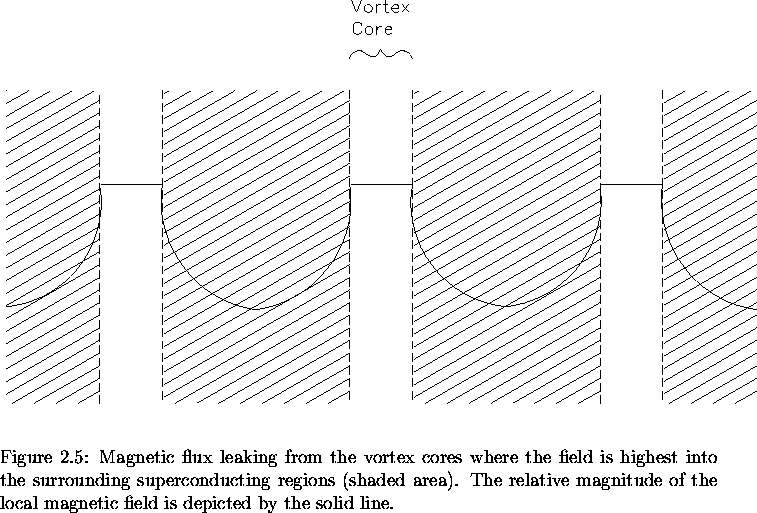In the dirty limit (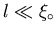), one can write the upper critcal field as :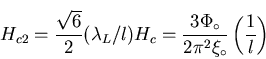(24)

Thus it is clear that increasing the impurity content of the superconductor and thus shortening the mean free path l results in a higher value of Hc2 for the material.

One may wonder how London's magnetic penetration depth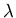could be incorporated into a theory for type-II superconductors. Although London's original theory is indeed valid for, it makes no mention of an upper critical field Hc2 (T). Nevertheless, one could anticipate some extension of the London model applicable to type-II superconductors by consideration of specific features. To start with, F. London theoretically predicted that magnetic flux is quantized in a superconducting annulus. An analogous treatment of the supercurrents that shield the vortex cores, leads one to deduce in several different ways [13,17], that the flux associated with each vortex is quantized and defined by: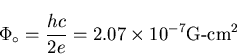(25)

where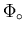is called the flux quantum, h is Planck's constant and c is the speed of light. London's original calculation of the flux quantumwas off by a factor of 2. The reason for this was his unknown knowledge of Cooper pairs. Experiments done on superconducting rings verify Eq. (2.25) as the correct equation .

For an isolated vortex line, the field as determined in the extreme type-II limit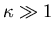is :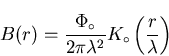(26)

where r is the distance from the axis of the vortex line, and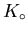denotes a modified Bessel function of the second kind. For small distances (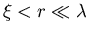), from the vortex-line axis, Eq. (2.26) reduces to :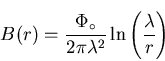(27)

while further away ():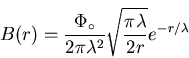(28)

The interaction between vortex lines becomes significant when their separation is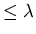. In a type-II superconductor, the local magnetic field outside a vortex core decays in an exponential manner so that one is justified in describing the field in this region by the London equations. In a crude sense, the mixed state of a type-II superconductor may be visualized as a type-I superconductor with a regular array of normal state regions (i.e. the vortex cores) embedded in its interior. The magnetic penetration depthis then a measure of the length over which magnetic flux leaks into the superconducting regions that encompass the vortex cores.

The existence of the mixed state can be argued through free energy considerations. Consider a sample in an applied magnetic field Happlied with a normal phase and a superconducting phase separated by a single interface. Realistically, the changeover between the two phases is not abrupt. Magnetic field leaks into the superconducting region a distance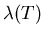, resulting in a contribution to the free surface energy of the interface that is negative. In addition, the order parameterdecreases to zero over a distance, thereby decreasing the actual volume of the sample which superconducts. This leads to a positive-energy contribution at the interface. The sum of both phenomena give the net surface energy per unit length at the interface as :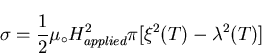(29)

A type-I superconductor where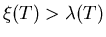, has positive surface-energy; while type-II superconductors with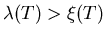, have negative surface-energy. A more precise calculation in the context of GL-theory predicts that the sign of the surface energy changes at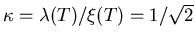. For a type-I superconductor in an applied field, the presence of normal-superconducting interfaces would increase the total free energy density because of positive surface-energy contributions. This situation is of course most unfavourable. For this reason, in the intermediate state of a type-I superconductor large normal regions will be formed which contain many flux quanta . On the other hand, in the case of a type-II superconductor, negative surface-energy contributions from normal-superconducting interfaces would reduce the free energy density. Consequently, the total energy is minimized by introducing as many of these interfaces as possible. This is exactly what happens in the mixed state of a type-II superconductor.

It has been argued that the vortex model borne out of Abrikosov's solution of the Ginzburg-Landau equations is the most energetically favourable form for the magnetic flux to assume inside the superconductor . Maximizing the surface-to-volume ratio of the normal regions leads to the lowest energy situation in the mixed state, because this provides the smallest positive surface-energy contribution. To achieve this, one can form normal regions which constitute either lamina of very small thickness (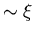) or alternatively, filaments of small diameter (). Theoretical calculations indicate that the latter formation is lowest in energy for.

Since Abrikosov's original prediction of a square vortex lattice, subsequent solutions of the GL equations for magnetic fields just below the upper critical field Hc2 (T), have convincingly shown that a periodic triangular array of vortex lines has the lowest free energy of all possible periodic solutions, and hence is the most stable configuration [13,40,41]. Decoration experiments which utilize small iron particles to make the vortices visible through an electron microscope have not only provided the best confirmation of the triangular arrangement but have also verified the flux quantum of Eq. (2.25) . It is clear from such experiments which verify the flux quantum that it is energetically more favourable to increase the number of vortices as one increases the magnetic field, rather than increasing the amount of flux in each vortex . Thus in an isotropic type-II superconductor, the vortex lines repel one another as a result of the magnetic force between them, to form an equilateral triangular lattice in accordance with energy minimization.Next: The Pairing Mechanism Up: Magnetic Properties of Conventional Superconductors Previous: BCS Theory for Conventional Superconductors
Jess H. Brewer
2001-09-28